Next: Algebraic Projective Geometry Up: Linear Approaches Previous: Linear Approaches

## Perspective Camera Models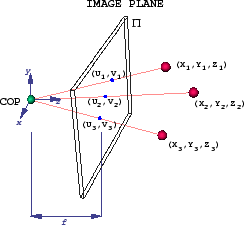Most Structure from Motion (linear and non-linear) techniques begin by assuming a perspective projection model as shown in Figure 3 which can be traced back to Durer and Renaissance painters. Alternative projection models include paraperspective or orthographic cases. Here, three 3D feature points are projecting onto an image plane (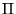) with perspective rays originating at the center of projection (COP), which would lie within the physical camera. The origin of the coordinate system is traditionally taken to be the COP and the focal length, f is the distance from the COP to the image plane along the principal axis (or optical axis). The optical axis is traditionally aligned with the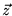axis. The projection of the COP onto the image plane along the optical axis is called the principal point.

Applying Thales theorem, we obtain the perspective projection formula as in Equation 1. Typically, the focal length f is set to 1 to simplify the expression since, in this model, f only varies the scaling of the image.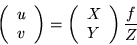(1)

This perspective projection is often referred to as a pinhole camera. Although the focal length is the most emphasized internal camera geometry parameter, there exist more complex full parameterizations. In fact, real cameras have many other internal geometry variables. A more complete camera parameterization is shown in Equation 2 . Here, the K matrix includes sx and sy, the scalings of the image plane along the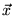and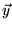axes. Also note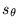the skew between theandaxes and (u0,v0) the coordinates of the principal point in the image plane. In addition to the linear effects summarized in the K matrix, there are other nonlinear and second order effects such as lens distortion. Typically, though, these second-order effects and even variables in K can be approximated and compensated for via standard corrective warping techniques .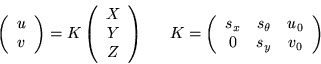(2)Next: Algebraic Projective Geometry Up: Linear Approaches Previous: Linear Approaches

1999-05-17